Finding Inverse of a matrix

Chapter 4 Class 12 Determinants
Concept wise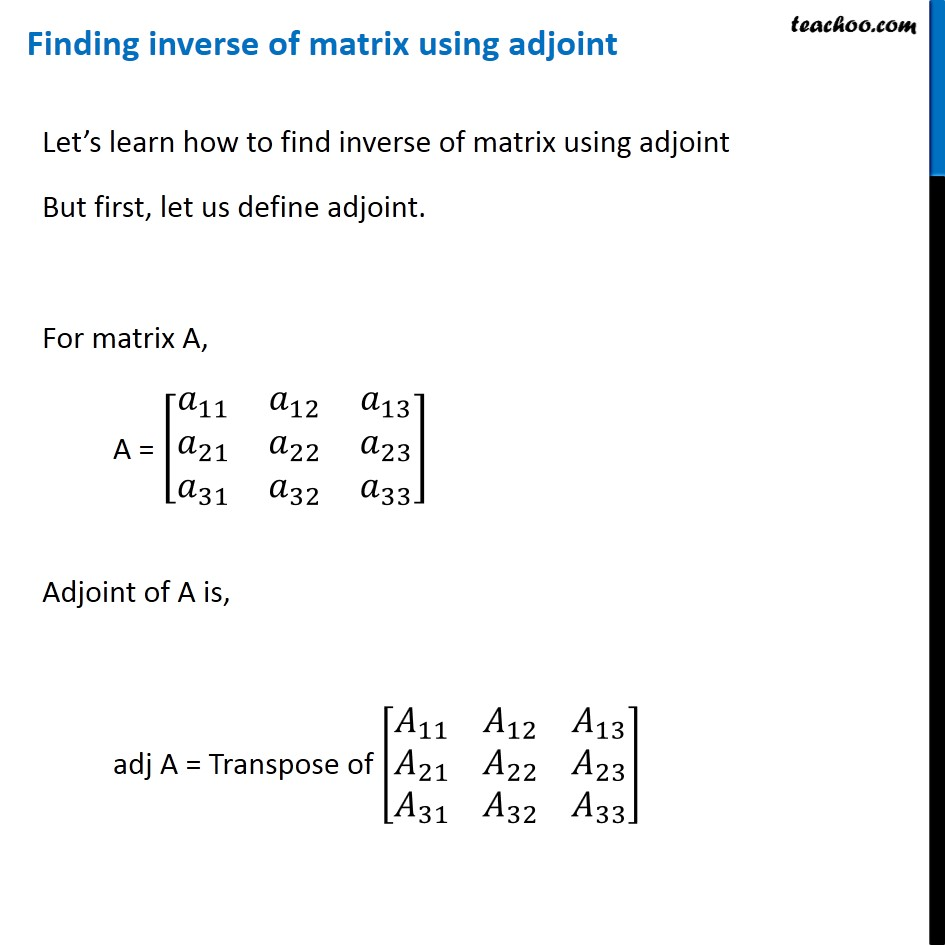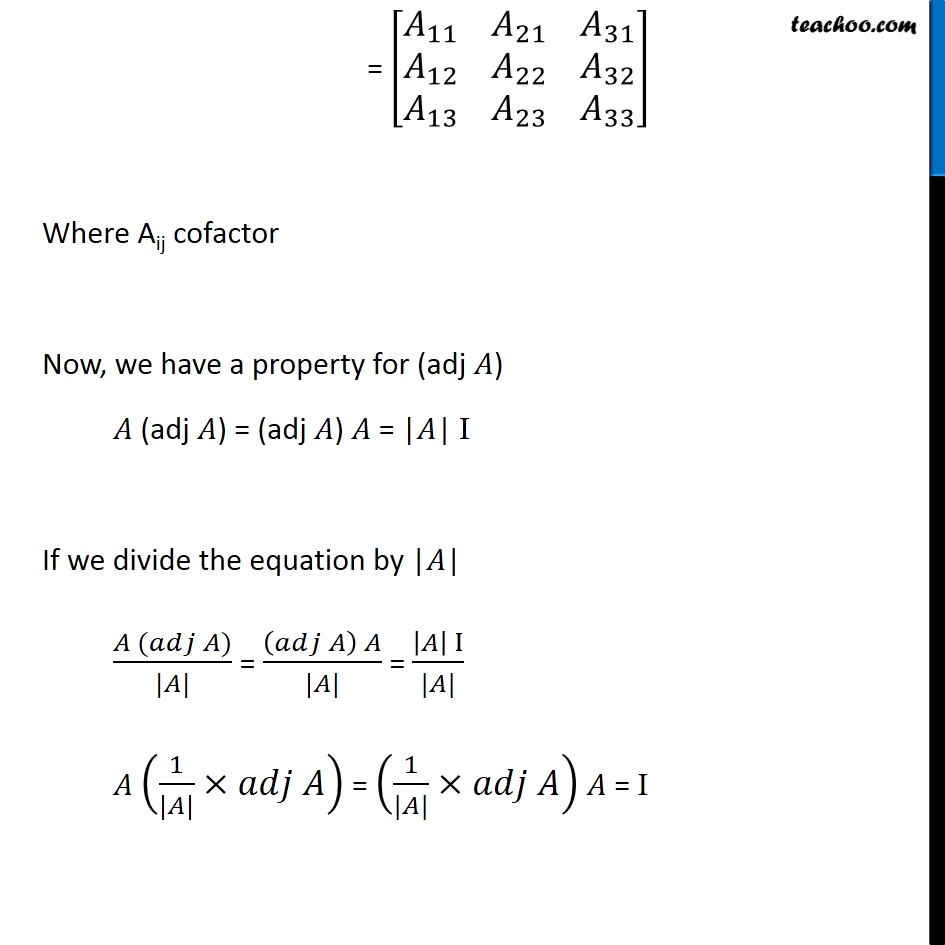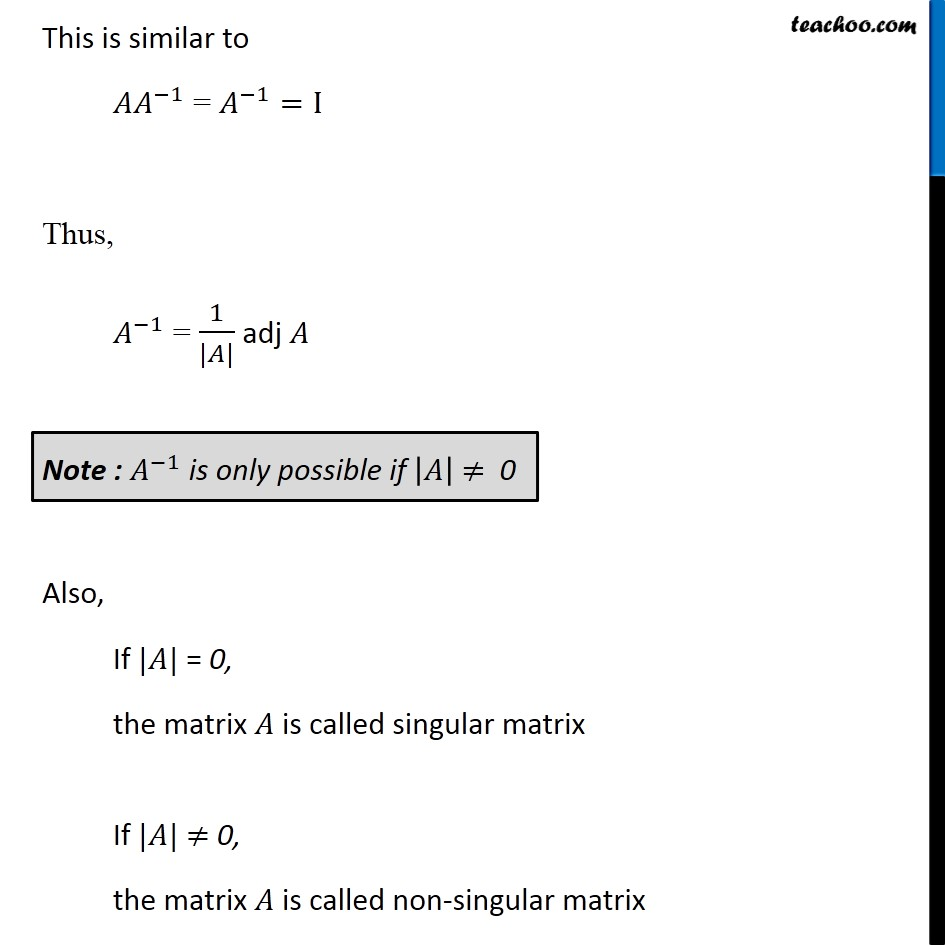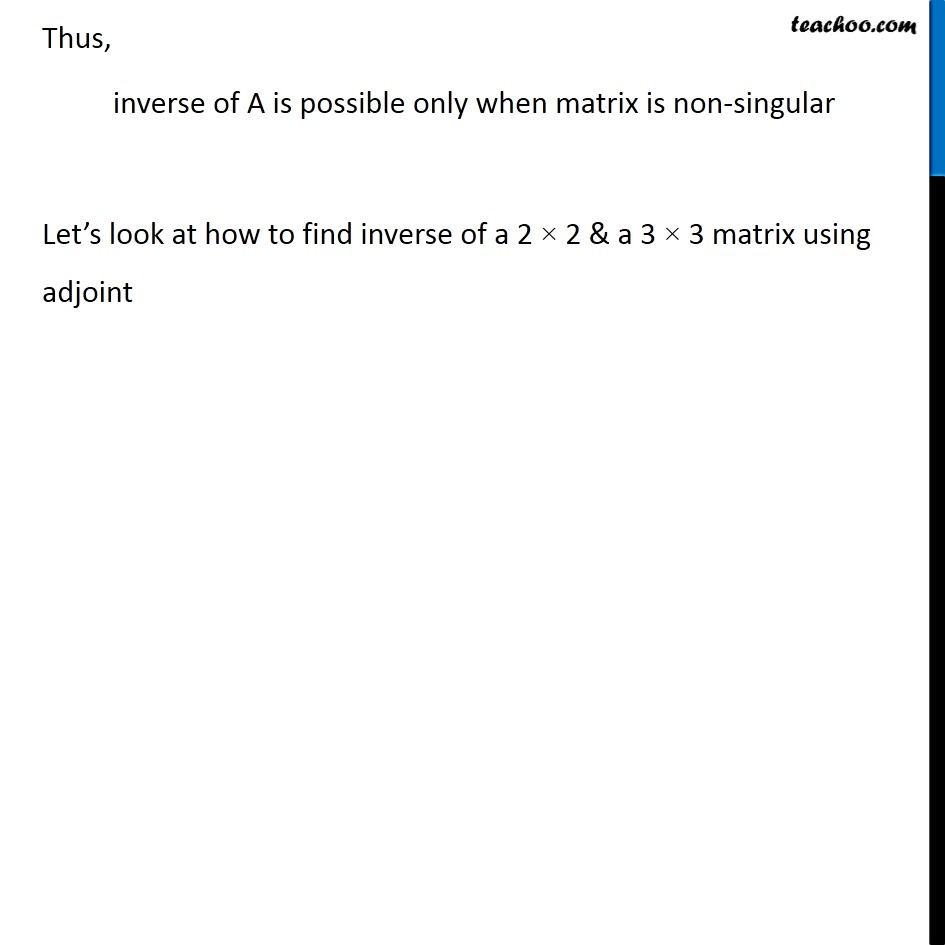## Inverse of a 2 × 2 matrix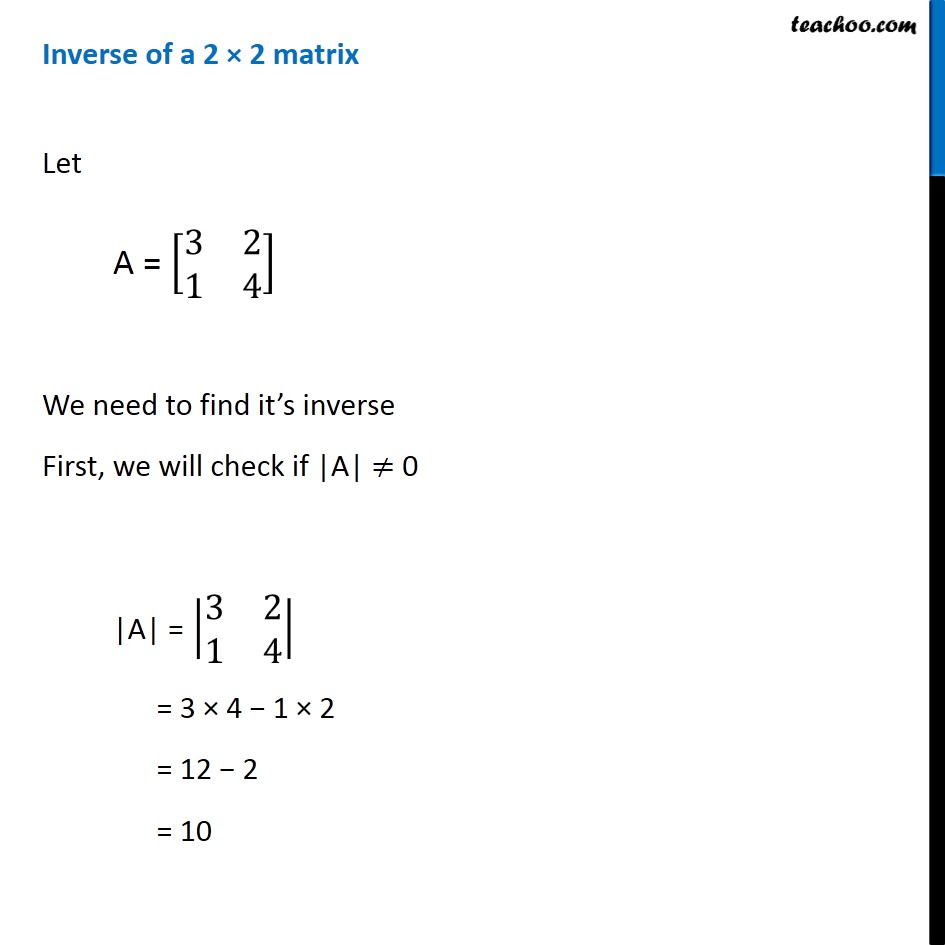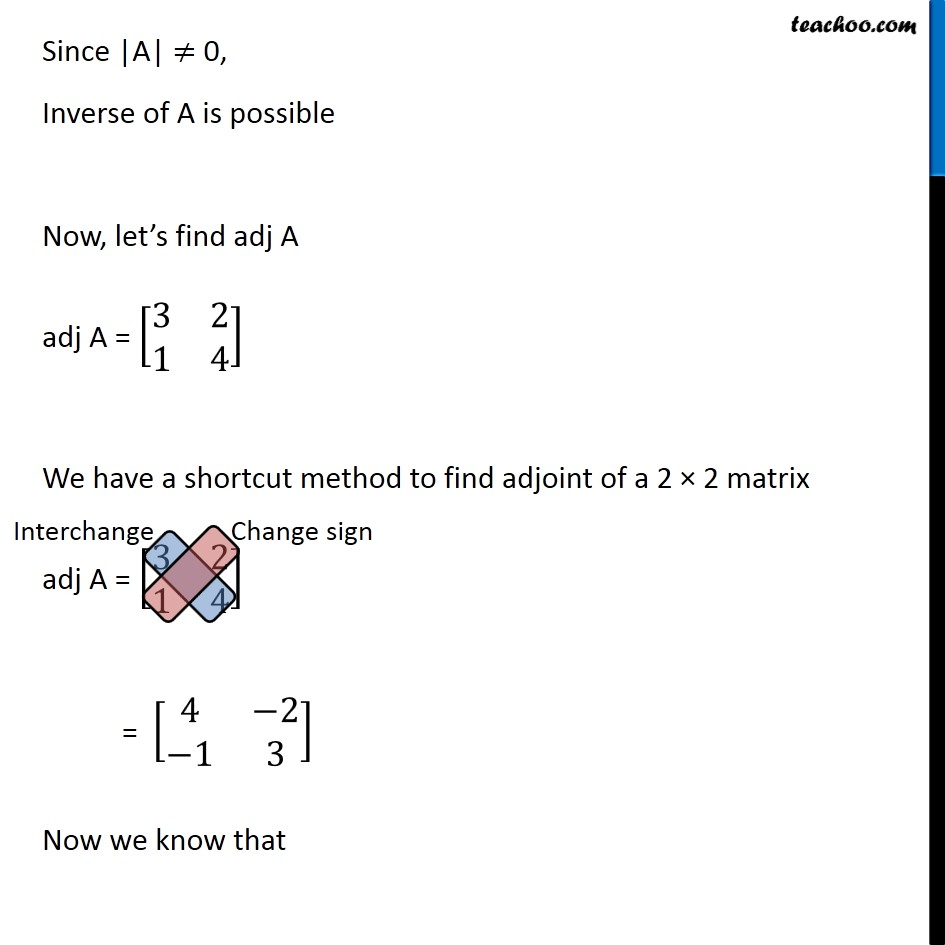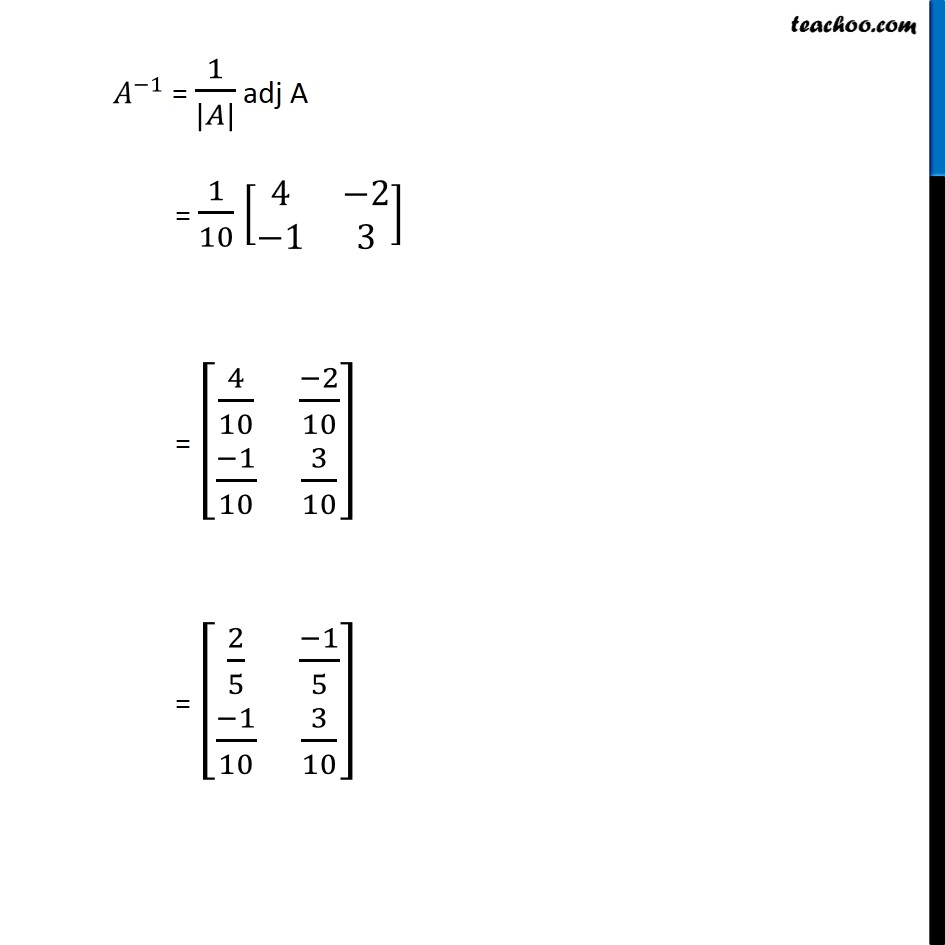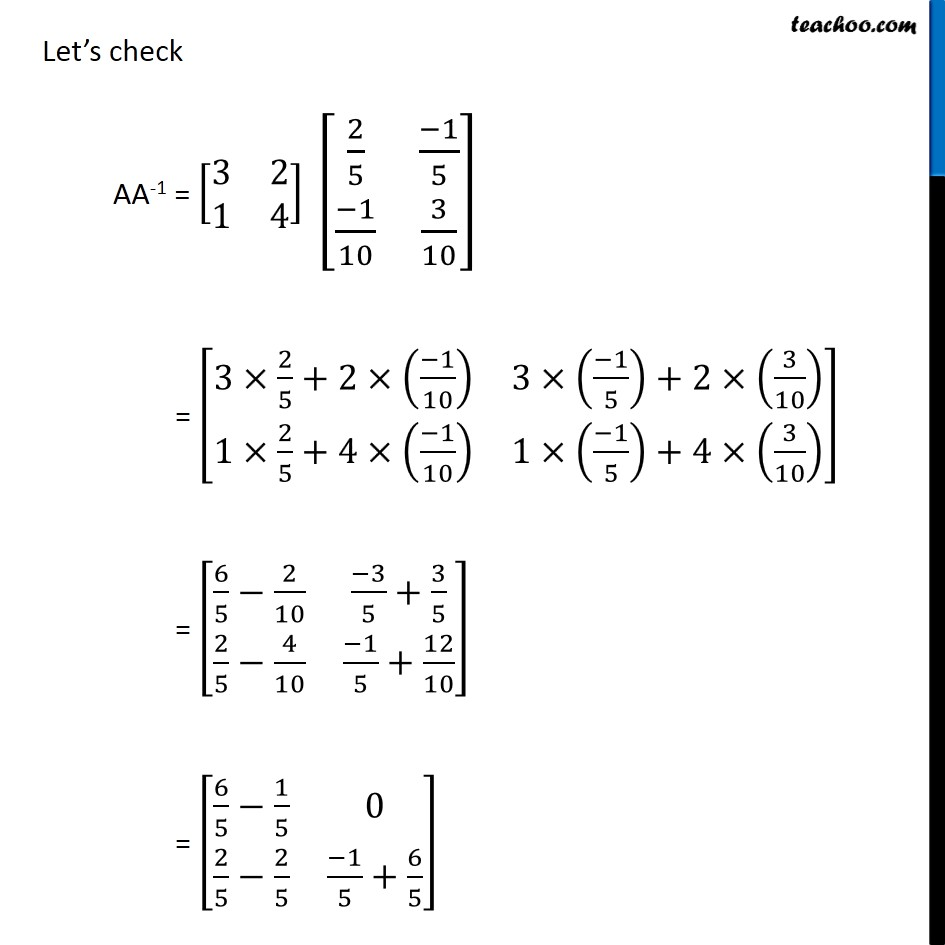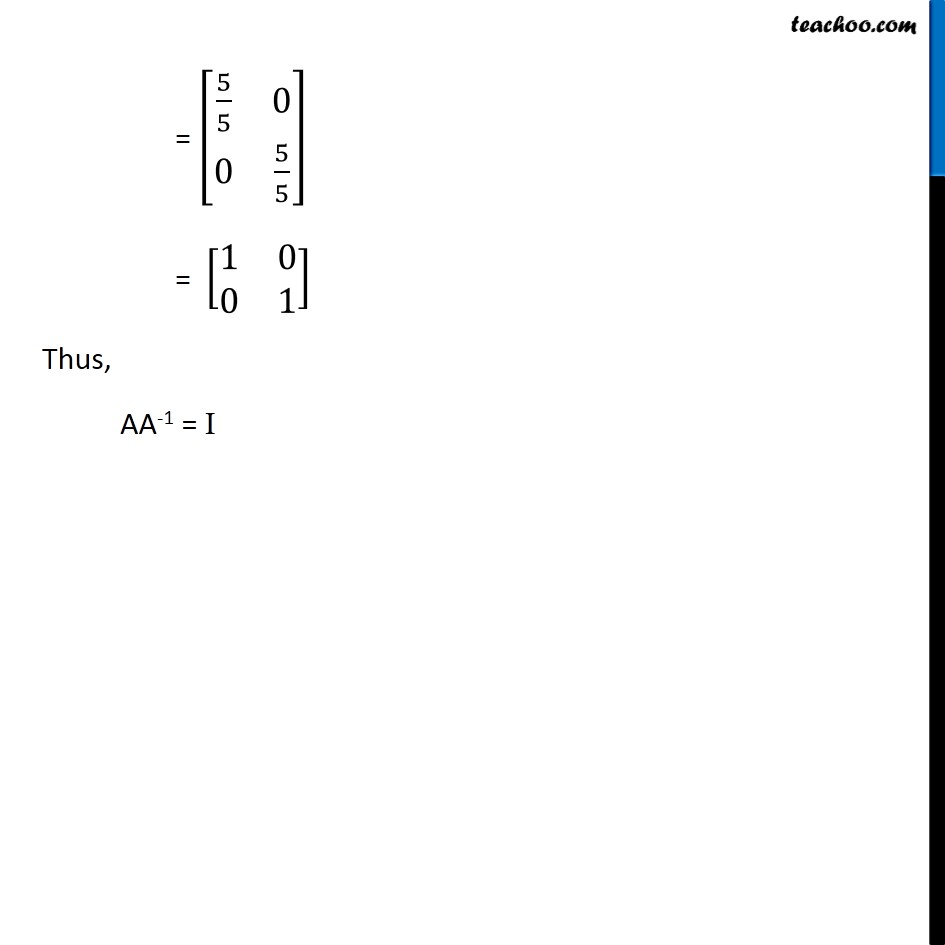## Inverse of a 3 × 3 matrix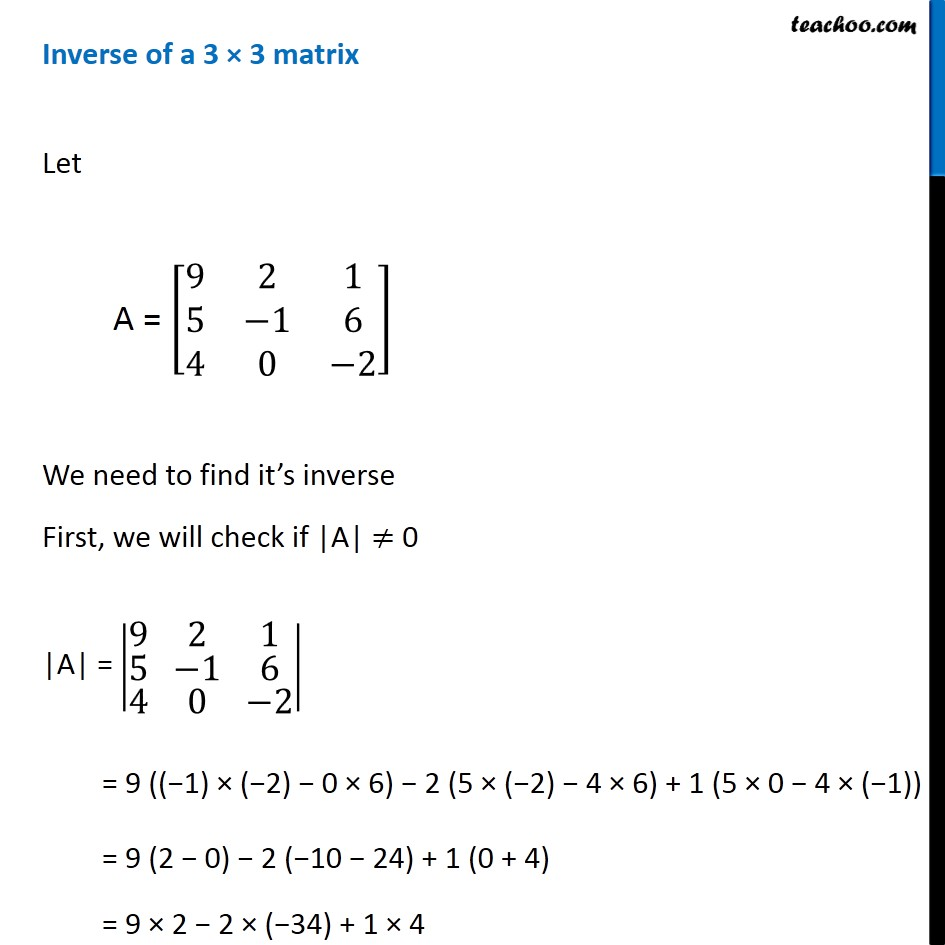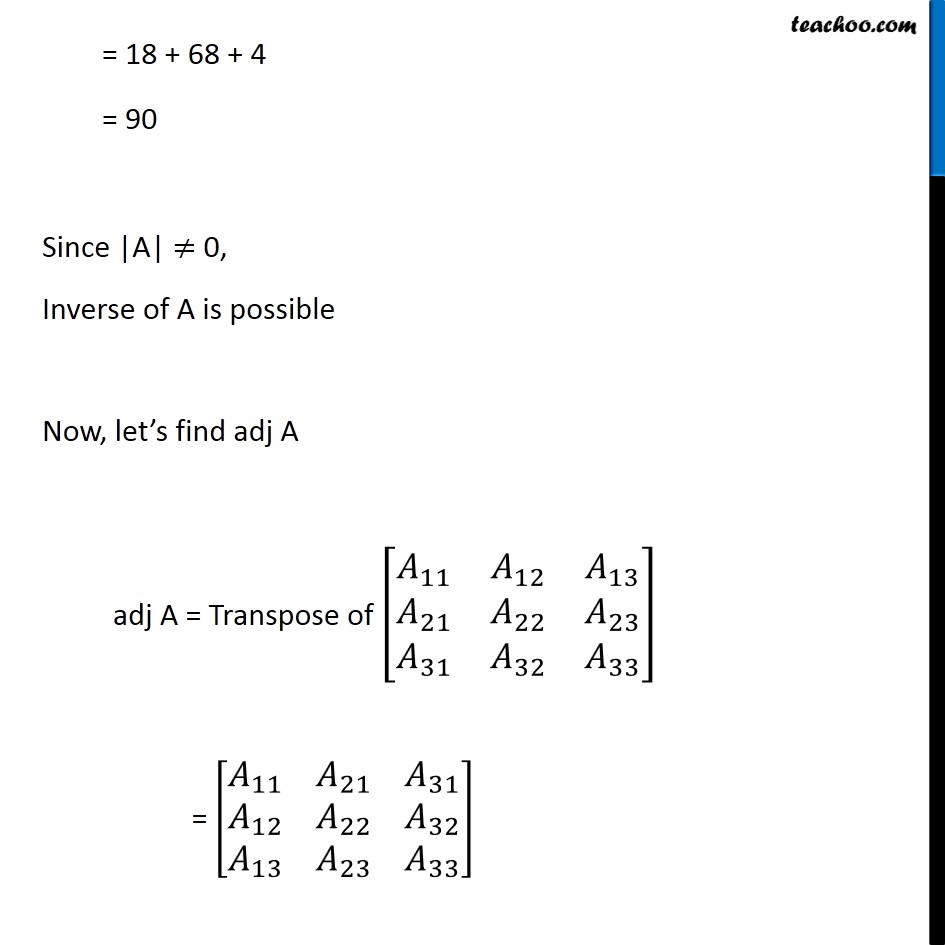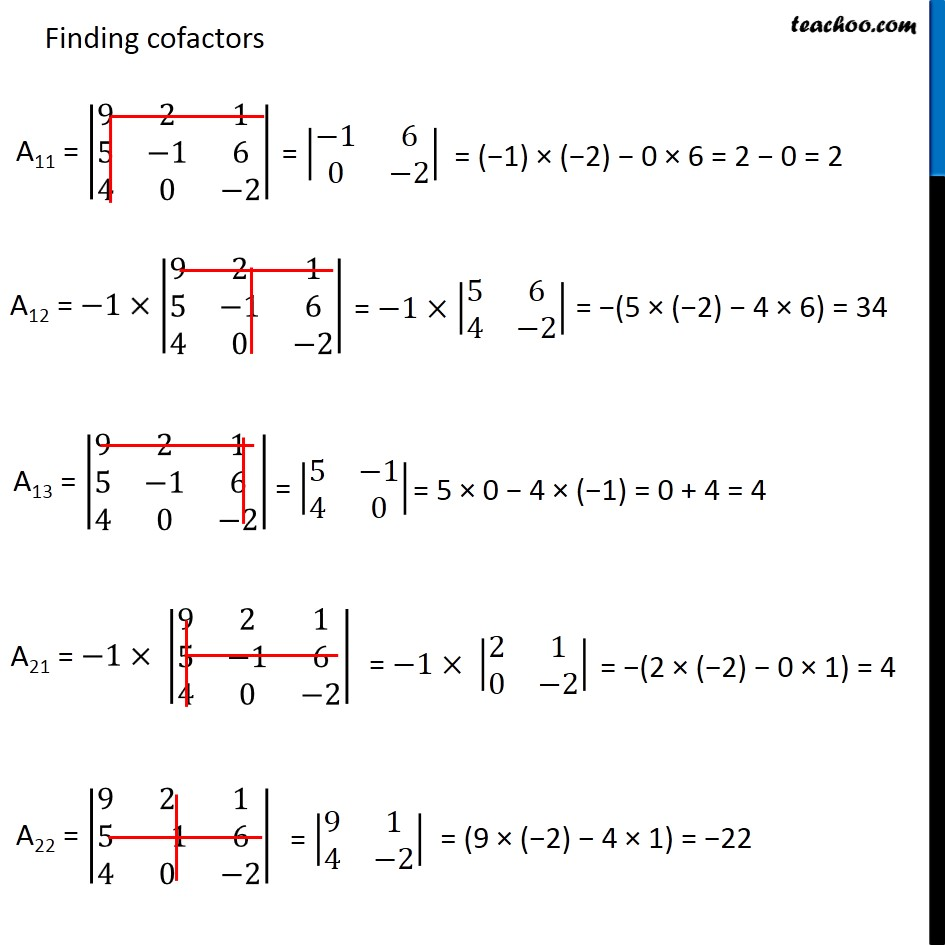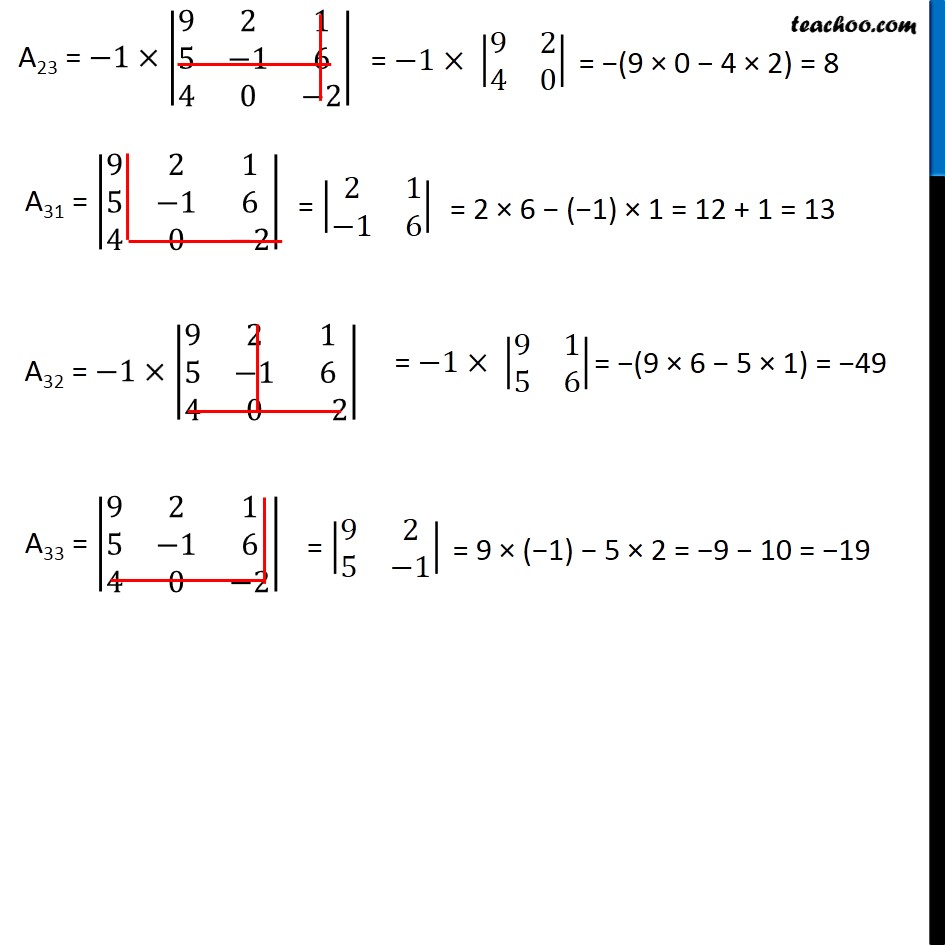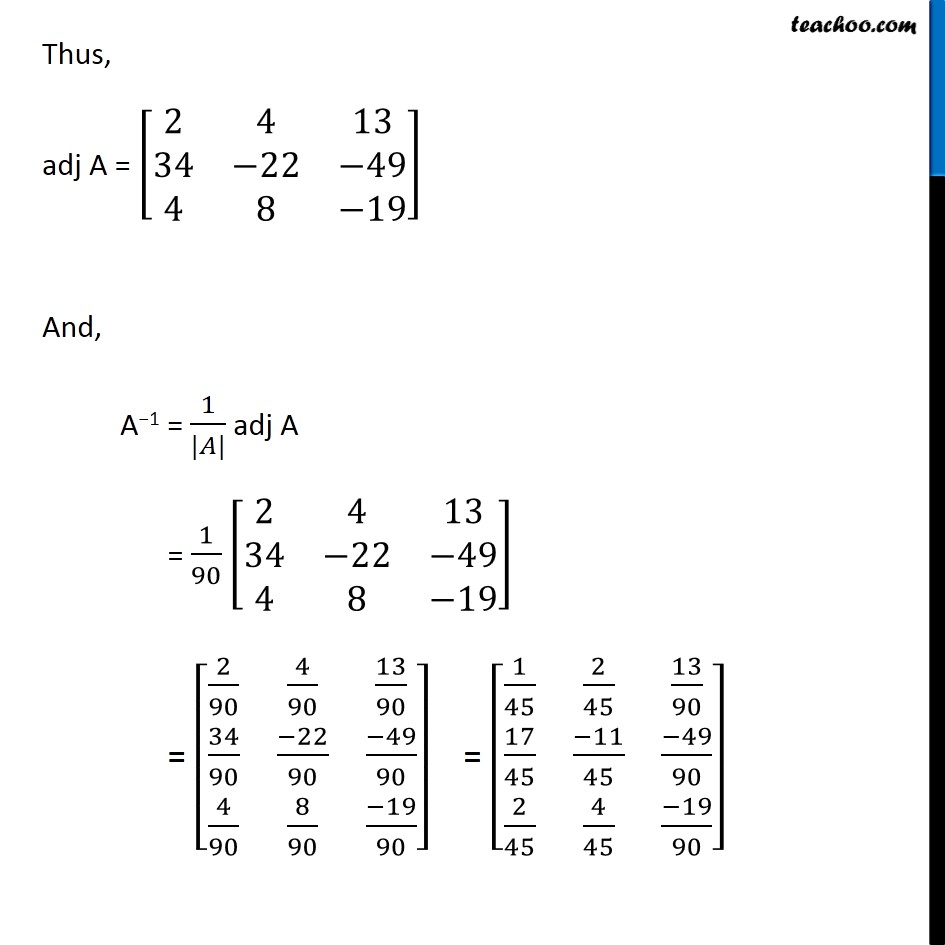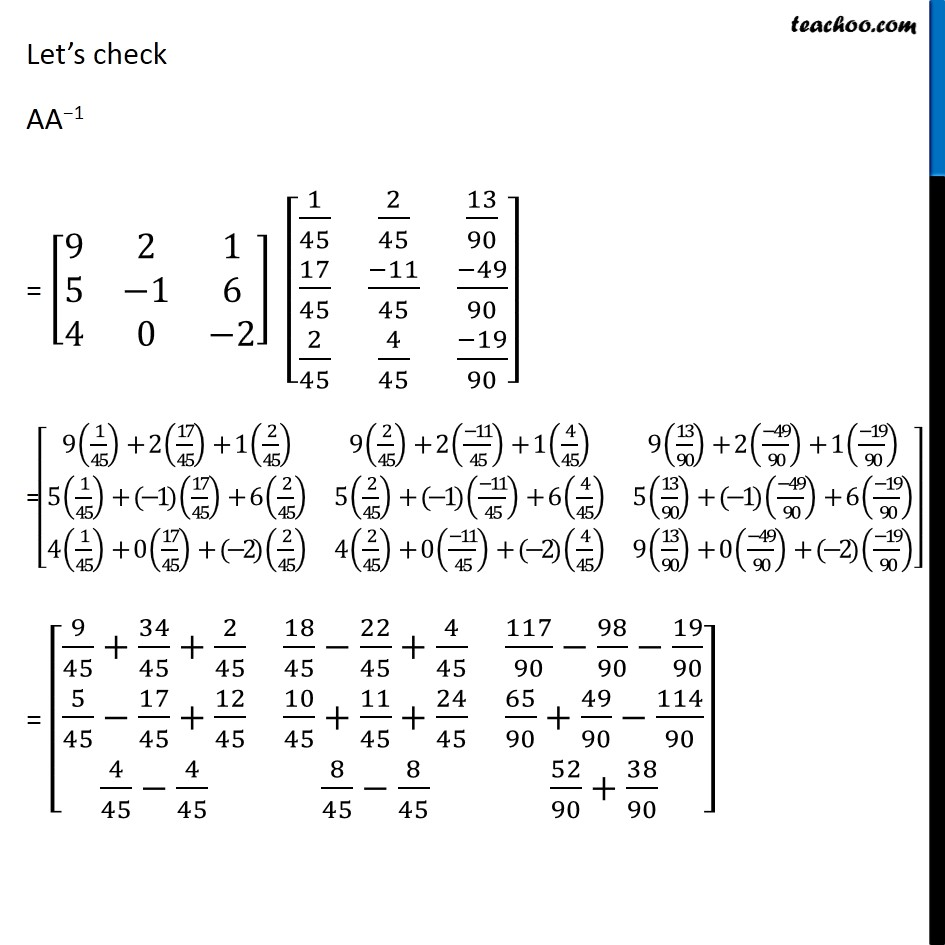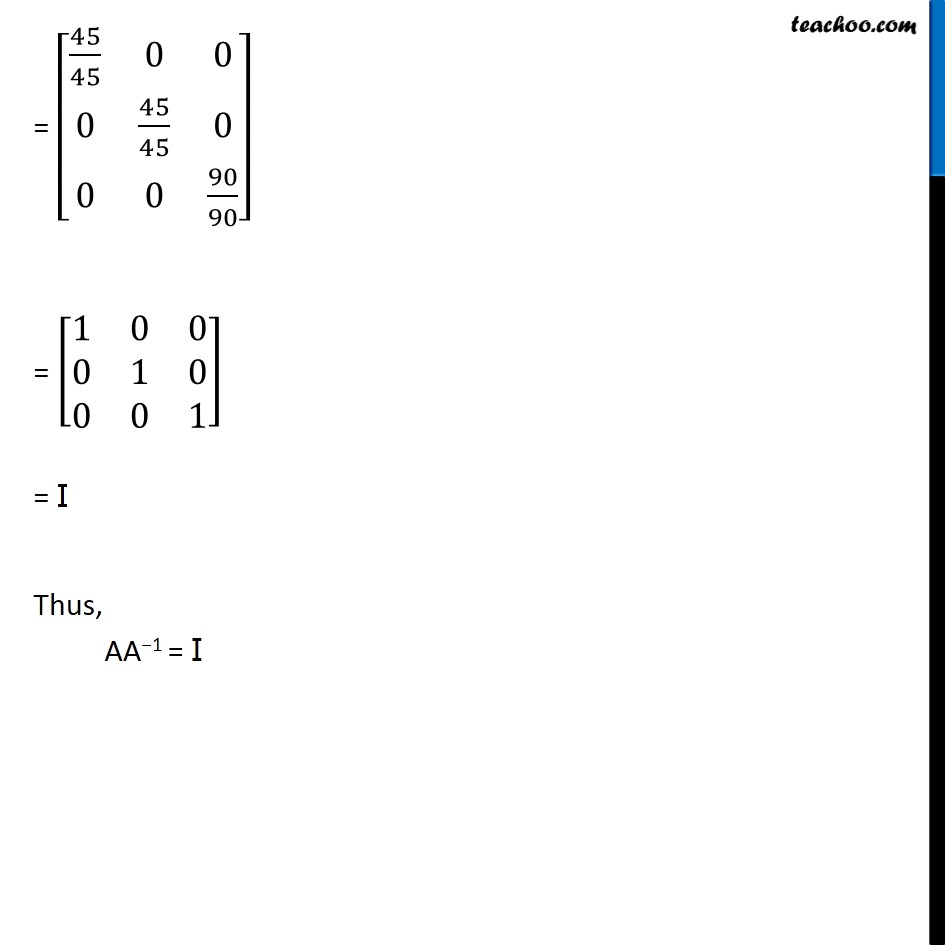Solve all your doubts with Teachoo Black (new monthly pack available now!)

### Transcript

Finding inverse of matrix using adjoint Let’s learn how to find inverse of matrix using adjoint But first, let us define adjoint. For matrix A, A = [■8(𝑎_11&𝑎_12&𝑎_13@𝑎_21&𝑎_22&𝑎_23@𝑎_31&𝑎_32&𝑎_33 )] Adjoint of A is, adj A = Transpose of [■8(𝐴_11&𝐴_12&𝐴_13@𝐴_21&𝐴_22&𝐴_23@𝐴_31&𝐴_32&𝐴_33 )] = [■8(𝐴_11&𝐴_21&𝐴_31@𝐴_12&𝐴_22&𝐴_32@𝐴_13&𝐴_23&𝐴_33 )] Where Aij cofactor Now, we have a property for (adj 𝐴) 𝐴 (adj 𝐴) = (adj 𝐴) 𝐴 = |𝐴| I If we divide the equation by |𝐴| (𝐴 (𝑎𝑑𝑗 𝐴))/(|𝐴|) = ((𝑎𝑑𝑗 𝐴) 𝐴)/(|𝐴|) = (|𝐴| I)/(|𝐴|) 𝐴 (1/(|𝐴|)×𝑎𝑑𝑗 𝐴) = (1/(|𝐴|)×𝑎𝑑𝑗 𝐴) 𝐴 = I This is similar to 〖𝐴𝐴〗^(−1) = 𝐴^(−1)=I Thus, 𝐴^(−1) = 1/(|𝐴|) adj 𝐴 Also, If |𝐴| = 0, the matrix 𝐴 is called singular matrix If |𝐴| ≠ 0, the matrix 𝐴 is called non-singular matrix Note : 𝐴^(−1) is only possible if |𝐴| ≠ 0 Thus, inverse of A is possible only when matrix is non-singular Let’s look at how to find inverse of a 2 × 2 & a 3 × 3 matrix using adjoint Inverse of a 2 × 2 matrix Let A = [■8(3&2@1&4)] We need to find it’s inverse First, we will check if |A| ≠ 0 |A| = |■8(3&2@1&4)| = 3 × 4 − 1 × 2 = 12 − 2 = 10 Since |A| ≠ 0, Inverse of A is possible Now, let’s find adj A adj A = [■8(3&2@1&4)] We have a shortcut method to find adjoint of a 2 × 2 matrix adj A = [■8(3&2@1&4)] = [■8(4&−2@−1&3)] Now we know that 𝐴^(−1) = 1/(|𝐴|) adj A = 1/10 [■8(4&−2@−1&3)] = [■8(4/10&(−2)/10@(−1)/10&3/10)] = [■8(2/5&(−1)/5@(−1)/10&3/10)] Let’s check AA-1 = [■8(3&2@1&4)] [■8(2/5&(−1)/5@(−1)/10&3/10)] = [■8(3×2/5+2×((−1)/10)&3×((−1)/5)+2×(3/10)@1×2/5+4×((−1)/10)&1×((−1)/5)+4×(3/10) )] = [■8(6/5−2/10&(−3)/5+3/5@2/5−4/10&(−1)/5+12/10)] = [■8(6/5−1/5&0@2/5−2/5&(−1)/5+6/5)] = [■8(5/5&0@0&5/5)] = [■8(1&0@0&1)] Thus, AA-1 = I Inverse of a 3 × 3 matrix Let A = [■8(9&2&1@5&−1&6@4&0&−2)] We need to find it’s inverse First, we will check if |A| ≠ 0 |A| = |■8(9&2&1@5&−1&6@4&0&−2)| = 9 ((−1) × (−2) − 0 × 6) − 2 (5 × (−2) − 4 × 6) + 1 (5 × 0 − 4 × (−1)) = 9 (2 − 0) − 2 (−10 − 24) + 1 (0 + 4) = 9 × 2 − 2 × (−34) + 1 × 4 = 18 + 68 + 4 = 90 Since |A| ≠ 0, Inverse of A is possible Now, let’s find adj A adj A = Transpose of [■8(𝐴_11&𝐴_12&𝐴_13@𝐴_21&𝐴_22&𝐴_23@𝐴_31&𝐴_32&𝐴_33 )] = [■8(𝐴_11&𝐴_21&𝐴_31@𝐴_12&𝐴_22&𝐴_32@𝐴_13&𝐴_23&𝐴_33 )] Finding cofactors A11 = |■8(9&2&1@5&−1&6@4&0&−2)| = (−1) × (−2) − 0 × 6 = 2 − 0 = 2 A12 = −1×|■8(9&2&1@5&−1&6@4&0&−2)| = −1× |■8(5&6@4&−2)| = −(5 × (−2) − 4 × 6) = 34 A13 = |■8(9&2&1@5&−1&6@4&0&−2)| = 5 × 0 − 4 × (−1) = 0 + 4 = 4 A21 = −1× |■8(9&2&1@5&−1&6@4&0&−2)| = −(2 × (−2) − 0 × 1) = 4 A22 = |■8(9&2&1@5&−1&6@4&0&−2)| = (9 × (−2) − 4 × 1) = −22 A23 = −1×|■8(9&2&1@5&−1&6@4&0&−2)| = −1× |■8(9&2@4&0)| = −(9 × 0 − 4 × 2) = 8 A31 = |■8(9&2&1@5&−1&6@4&0&−2)| = |■8(2&1@−1&6)| = 2 × 6 − (−1) × 1 = 12 + 1 = 13 A32 = −1×|■8(9&2&1@5&−1&6@4&0&−2)| = −1× |■8(9&1@5&6)| = −(9 × 6 − 5 × 1) = −49 A33 = |■8(9&2&1@5&−1&6@4&0&−2)| = |■8(9&2@5&−1)| = 9 × (−1) − 5 × 2 = −9 − 10 = −19 Thus, adj A = [■8(2&4&13@34&−22&−49@4&8&−19)] And, A−1 = 1/(|𝐴|) adj A = 1/90 [■8(2&4&13@34&−22&−49@4&8&−19)] = [■8(2/90&4/90&13/90@34/90&(−22)/90&(−49)/90@4/90&8/90&(−19)/90)] = [■8(1/45&2/45&13/90@17/45&(−11)/45&(−49)/90@2/45&4/45&(−19)/90)] Let’s check AA−1 = [■8(9&2&1@5&−1&6@4&0&−2)] [■8(1/45&2/45&13/90@17/45&(−11)/45&(−49)/90@2/45&4/45&(−19)/90)] = [■8(9(1/45)+2(17/45)+1(2/45)&9(2/45)+2((−11)/45)+1(4/45)&9(13/90)+2((−49)/90)+1((−19)/90)@5(1/45)+(−1)(17/45)+6(2/45)&5(2/45)+(−1)((−11)/45)+6(4/45)&5(13/90)+(−1)((−49)/90)+6((−19)/90)@4(1/45)+0(17/45)+(−2)(2/45)&4(2/45)+0((−11)/45)+(−2)(4/45)&9(13/90)+0((−49)/90)+(−2)((−19)/90) )] = [■8(9/45+34/45+2/45&18/45−22/45+4/45&117/90−98/90−19/90@5/45−17/45+12/45&10/45+11/45+24/45&65/90+49/90−114/90@4/45−4/45&8/45−8/45&52/90+38/90)] = [■8(45/45&0&0@0&45/45&0@0&0&90/90)] = [■8(1&0&0@0&1&0@0&0&1)] = I Thus, AA−1 = I